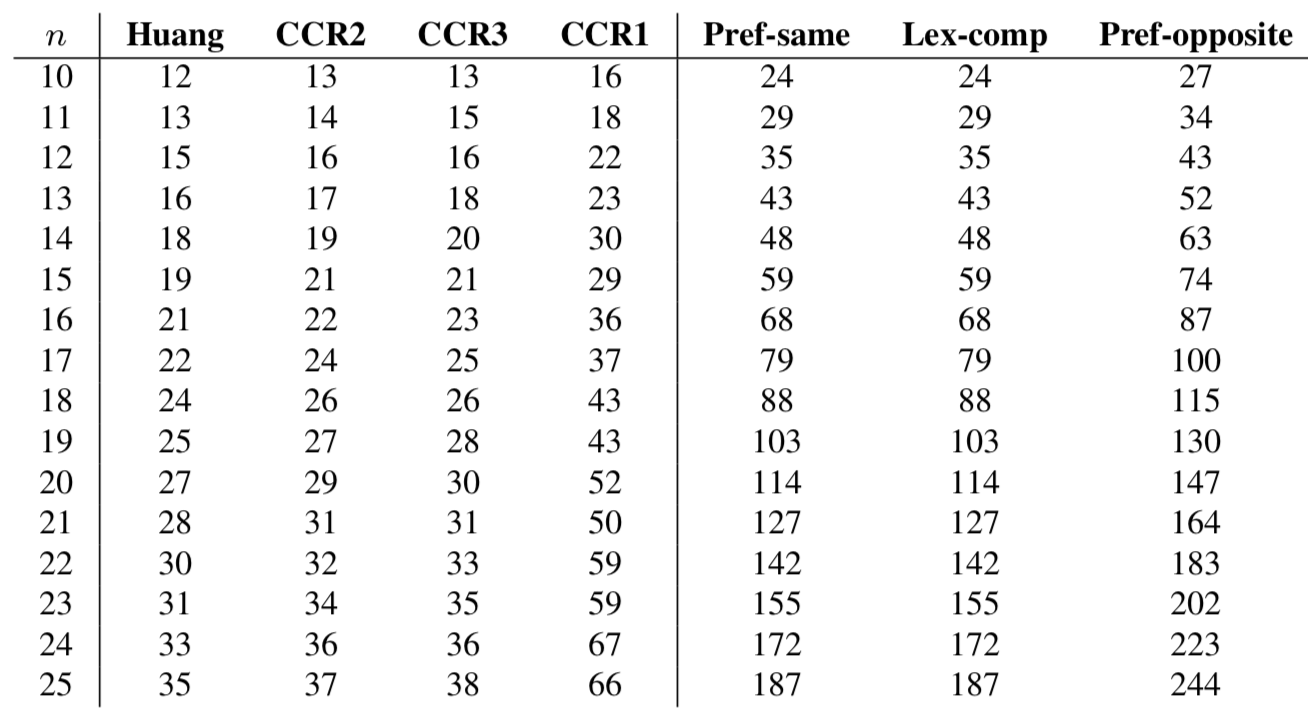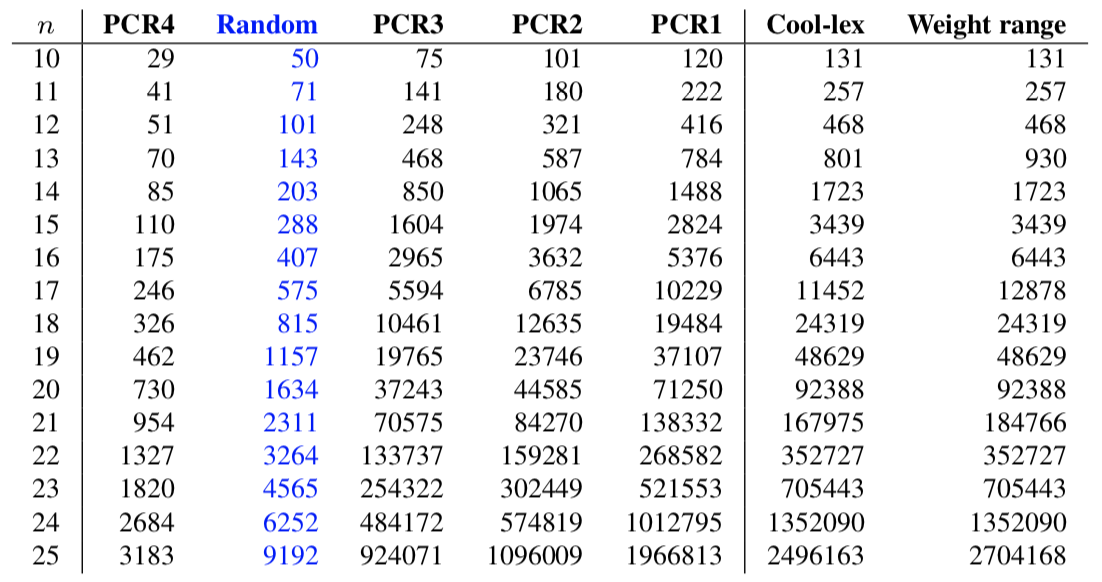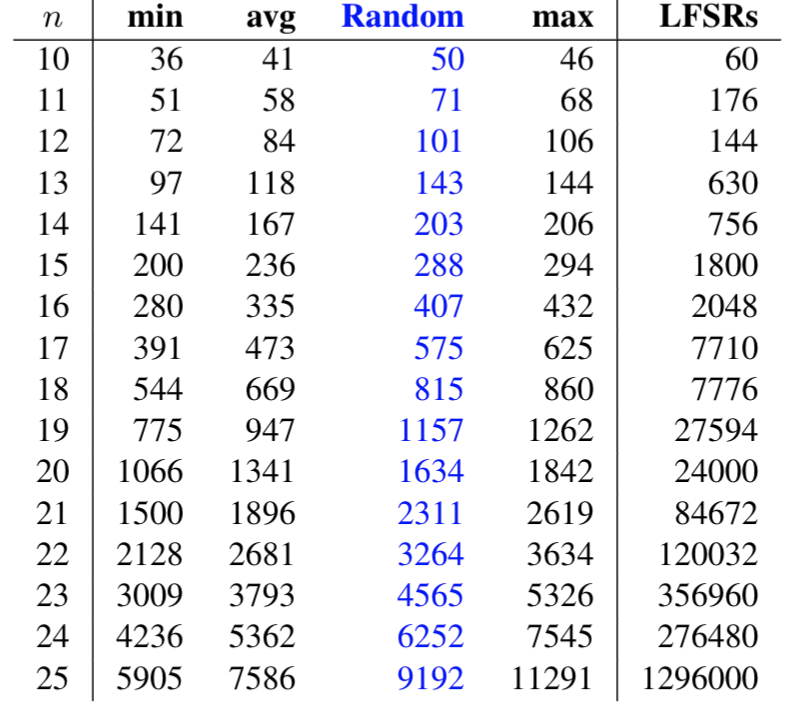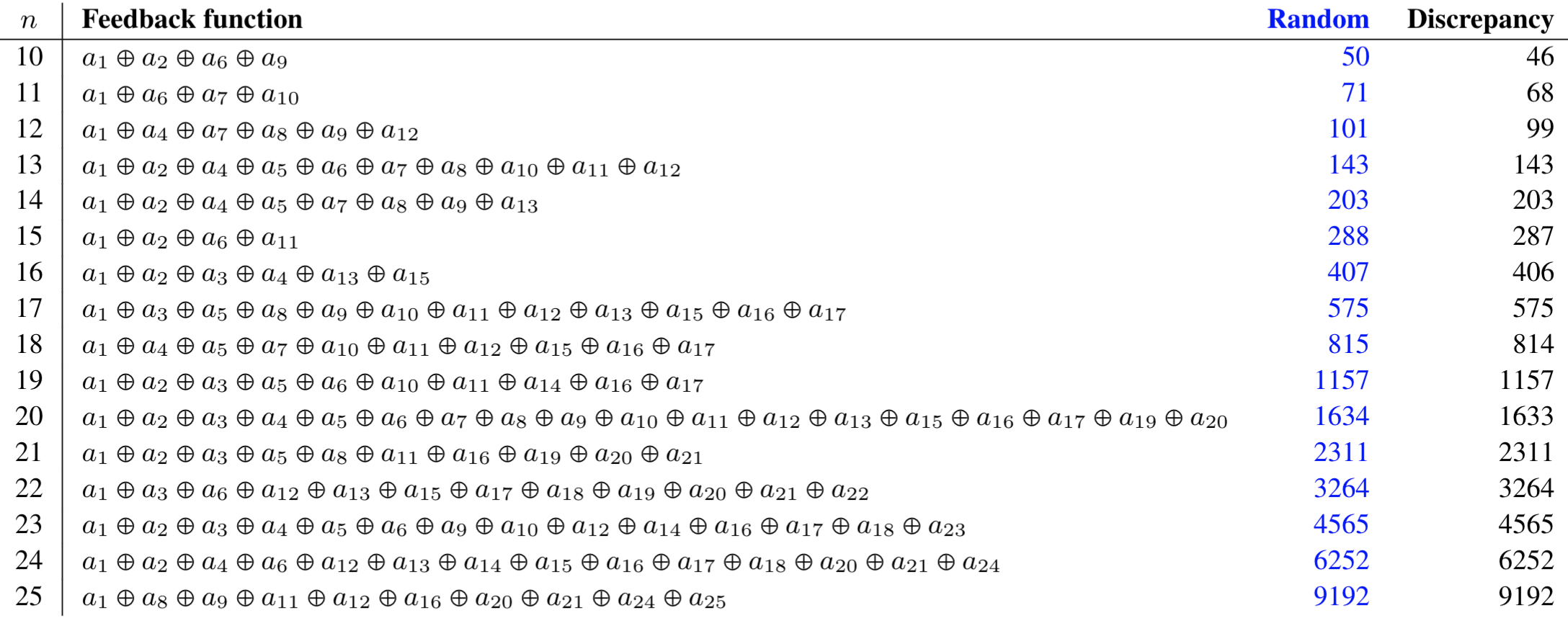#De Bruijn Sequence and Universal Cycle Constructions

Min/Random/Max Discrepancy Output
 Algorithm Min (Huang) Random (LFSR) Max (Weight range) Order $n$ 1 2 3 4 5 6 7 8 9 10 11 12 13 14 15 16 17 18 19 20 21 22 23 24 25
Properties of binary DB sequences

As articulated in [Gol17], binary DB sequences

• are balanced as they contain the same number of $0$s and $1$s;
• satisfy a run property as there are an equal number of contiguous runs of 0s and 1s of the same length in the sequence;
• satisfy a span-$n$ property as they contain every distinct length $n$ binary string as a substring.
Despite these properties, many DB sequences display other properties that are far from random. In the greedy Prefer-largest construction, as one would expect, the resulting DB sequence has more $1$s than $0$s at the start of the sequence. One measure that accounts for this is the discrepancy: the maximum absolute difference between the number of $0$s and $1$s in any substring of a given sequence. For instance, the discrepancy in the DB sequence $000000\underline{1111110111100111010111}000110110100110010110000101010001001$ is $|17-5|=12$ as witnessed by the underlined substring. Interestingly, of all the DB sequence constructions investigated, the sequences with discrepancy closest to a random sequence are those generated by LFSRs (see below). However, a DB sequence known to be generated by an LFSR can be completely determined after reading only $2n$ bits [Mas69].

#### Discrepancy

The DB sequences with the smallest discrepancy are based on the complementing cycling register (CCR). The two CCR concatenation constructions in [GS18], which correspond to the shift-rules CCR2 and CCR3, have discrepancy less than $2n$. This is because the maximum discrepancy in any single CCR cycle is $n$ [GS17]. Experimentally, the shift rule by Huang [H90] demonstrates slightly smaller discrepancy.

The greedy Prefer-same and Prefer-opposite, along with the lexicographic composition concatenation approach can all be generated via shift-rules based on the PRR. They all appear to have discrepancies of $O(n^2)$. The table below summarizes their discrepancies along with the four shift rules based on the CCR.The DB sequences with the maximal discrepancy can be obtained by considering weight (density) ranges. By taking a universal cycle $U_1$ for all binary strings with weight from 0 to $\lceil n/2 \rceil -1$ and joining it with a universal cycle $U_2$ for all binary strings with weights from $\lceil n/2 \rceil$ to $n$, the discrepancy will be close to the discrepancy of $U_1$, which is ${n-1 \choose \lfloor n/2 \rfloor}$. It is conjectured that the maximal discrepancy for any DB sequence is ${n-1 \choose \lfloor n/2 \rfloor} + \lfloor n/2 \rfloor$, which by Stirling's approximation is $\Theta(\frac{2^n}{\sqrt{n}})$. This is exactly the discrepancy obtained by this construction, which is available for generation and download above [GS20]. The table below shows the discrepancy of this sequence, along with the cool-lex concatenation construction which is also based on weight ranges (and the PSR/CSR). The table also illustrates the discrepancies for the shift rules PCR1 (Granddaddy), PCR2 (Grandmama), PCR3, and PCR4. The sequences generated by the PCR1 construction are known to have discrepancy $\Theta(\frac{2^n\log n}{n})$ [CH10].The "Random" column is obtained by taking the average discrepancy of 10000 random sequences of length $2^n$. Such a random sequence is expected to have discrepancy $\Theta(2^{n/2} \sqrt{\log n})$. It remains an interesting problem to define/construct a DB sequence that is provably close to these random values. Experimentally, the shift-rule PCR4 constructs a DB sequence that is closest to the expected discrepancy of a random sequence.

The table below considers the discrepancy over all LFSRs based on primitive polynomials. The minimum, average, and maximum discrepancies are illustrated against our random target. While these sequences demonstrate discrepancy closest to what one expects from a random sequence, the fact that the underlying LFSR can be so easily decoded (as noted above) limits their application.For $12< n \leq 25$, there exists a DB sequence generated by an LFSR (try it above!) that has discrepancy differing by at most one from the random target. Feedback functions for such LFSRs are given below.References

• [CH10] J. Cooper and C. Heitsch. The discrepancy of the lex-least de Bruijn sequence. Discrete Math., 310(6-7):1152-1159, 2010.

• [Gol17] S. W. Golomb. Shift Register Sequences (Third Edition). World Scientific, Singapore, 2017.

• [GS17] D. Gabric and J. Sawada. A de Bruijn sequence construction by concatenating cycles of the complemented cycling register. In Combinatorics on words, volume 10432 of Lecture Notes in Comput. Sci., pages 49-58. Springer, Cham, 2017.

• [GS18] D. Gabric and J. Sawada. Constructing de Bruijn sequences by concatenating smaller universal cycles. Theoret. Comput. Sci., 743:12-22, 2018.

• [GS20] D. Gabric and J. Sawada. Investigating the discrepancy property of de Bruijn sequences. Manuscript, 2020.

• [Mas69] J. Massey. Shift-register synthesis and BCH decoding. IEEE Transactions on Information Theory, 15(1):122–127,1969.